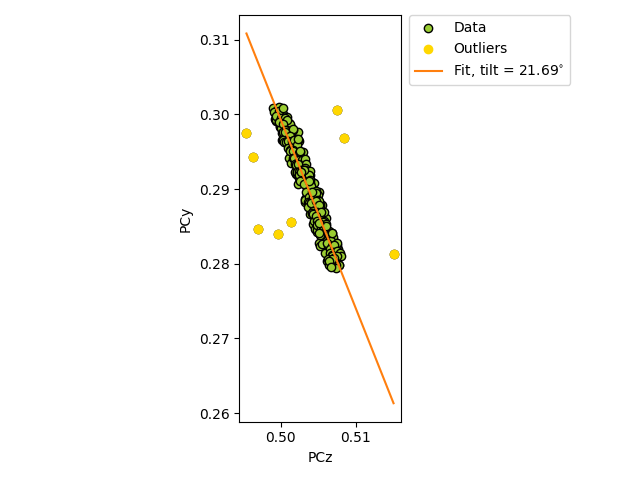# Estimate tilt about the detector x axis#

This example shows how to (robustly) estimate the tilt about the detector $$X_d$$ axis which brings the sample plane normal into coincidence with the detector plane normal (but in the opposite direction) .

The estimate is found using estimate_xtilt() which performs linear regression of pcz vs. pcy.

To test the estimation, we add some noise to realistic projection center (PC) values (PCx, PCy, PCz). The realistic PCs are extrapolated from a PC in the upper left corner of a map, assuming a nominal sample tilt of 70 degrees, a detector tilt of 0 degrees, a detector pixel size of 70 microns and a sample step size of 50 microns.True/estimated tilt about detector x [deg]: 20.00/21.53


import kikuchipy as kp
import numpy as np

# Create an initial detector with one PC assumed to be for the upper
# left corner of a map
det0 = kp.detectors.EBSDDetector(
shape=(480, 480),
pc=(0.5, 0.3, 0.5),
sample_tilt=70,
tilt=0,
px_size=70,
)

# Extrapolate a map of PCs
nav_shape = (15, 20)
det = det0.extrapolate_pc(
pc_indices=[0, 0],
step_sizes=(50, 50),
)

# Add +/- 0.0025 as random noise to PCy and PCz
rng = np.random.default_rng()

is_outlier[outlier_idx1d] = True
noise_outlier = rng.uniform(-0.01, 0.01, outlier_idx1d.size)
det.pcz[outlier_idx2d] += noise_outlier

# Robust estimation by detecting outliers
xtilt, detected_outliers = det.estimate_xtilt(
detect_outliers=True, degrees=True, return_outliers=True
)

# Print true tilt and estimated tilt
true_tilt = 90 - det.sample_tilt + det.tilt
print(f"True/estimated tilt about detector x [deg]: {true_tilt:.2f}/{xtilt:.2f}")

correct_outliers = np.isin(outlier_idx1d, np.where(detected_outliers))